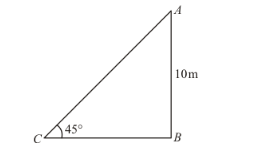# The height of a tower is 10 m.

Question:

The height of a tower is 10 m. What is the length of its shadow when Sun's altitude is 45°?

Solution:

Letbe the length of shadow ismGiven that: Height of tower ismeters and altitude of sun isHere we have to find length of shadow.

So we use trigonometric ratios.

In a triangle,

$\Rightarrow \tan C=\frac{A B}{B C}$

$\Rightarrow \tan 45^{\circ}=\frac{A B}{A C}$

$\Rightarrow 1=\frac{10}{x}$

$\Rightarrow x=10$

Hence the length of shadow is $10 \mathrm{~m}$.